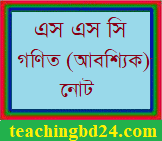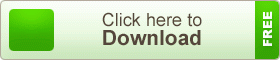# SSC Mathematics Note 12th Chapter Simple Simultaneous Equations with Two Variables

SSC Mathematics Note 12th Chapter Simple Simultaneous Equations with Two Variables. For solving the mathematical problems, the most important topic of  Algebra is the equation. In classes VI and VII, we have got the idea of the simple equation and have known how to sol. the simple equation with one variable. In class V. We have soled the simple simultaneous equations by the methods of substitution and elimination and by graphs.  We have also learned how to form and solve simple simultaneous equations related to real life,  problems.  In this chapter, the idea of simple simultaneous equations has been expanded and new methods of the solution have been discussed. Besides, in this chapter, solution by graphs and formation of simultaneous equations related to real life problems and their solutions have been discussed in detail.

## SSC Mathematics Note 12th Chapter Simple Simultaneous Equations with Two VariablesConveniently, one equation or both equations are multiplied by such a number so that after multiplication, the absolute value of the coefficients of the same variable become equal.  Then as per need,  if the equations are added or subtracted,  the variable with equal coefficient will be eliminated.

teachingbd24.com is such a website where you will get all kinds of necessary information regarding educational notes, suggestions and question patterns of schools, colleges, and madrasas. Particularly, you will get here special notes of physics that will be immensely useful to both students and teachers. The builder of the website is Mr. Md. Shah Jamal who has been serving for 32 years as an Assistant Professor of Physics at BAF Shaheen College Dhaka. He expects that this website will meet up all the needs of Bengali version learners /students. He has requested concerned students and teachers to spread this website home and abroad.